# Specifying a Class A class is a way

• Slides: 27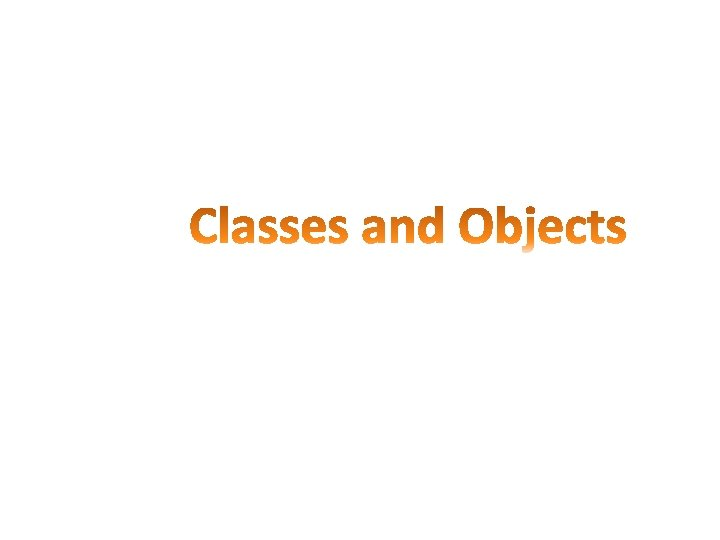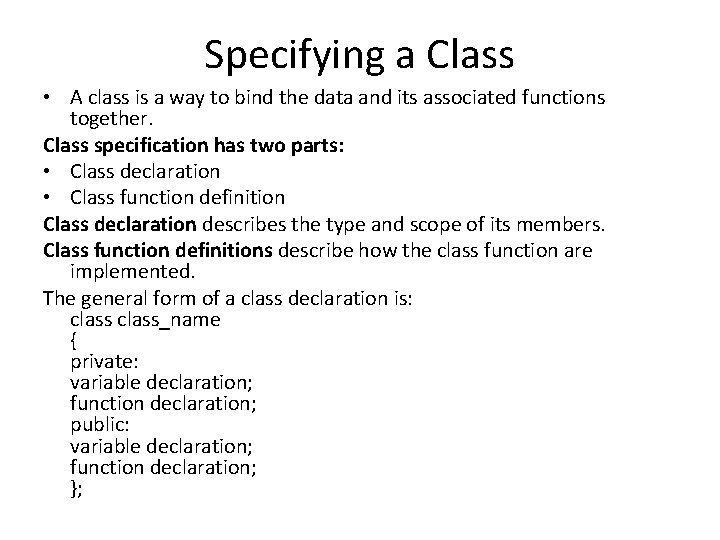Specifying a Class • A class is a way to bind the data and its associated functions together. Class specification has two parts: • Class declaration • Class function definition Class declaration describes the type and scope of its members. Class function definitions describe how the class function are implemented. The general form of a class declaration is: class_name { private: variable declaration; function declaration; public: variable declaration; function declaration; };A class definition starts with the keyword class followed by the class name; and the class body, enclosed by a pair of curly braces, terminated by a semicolon. • Functions and variable are called as class member. • Private and public are access modifier, known for visibility labels. These keyword are followed by a semicolon. • In a class by default all the data and functions member are private. • A public data and functions can be accessed from outside the class anywhere within the scope of the class object. • Private data and functions can only be accessed from within the class itself.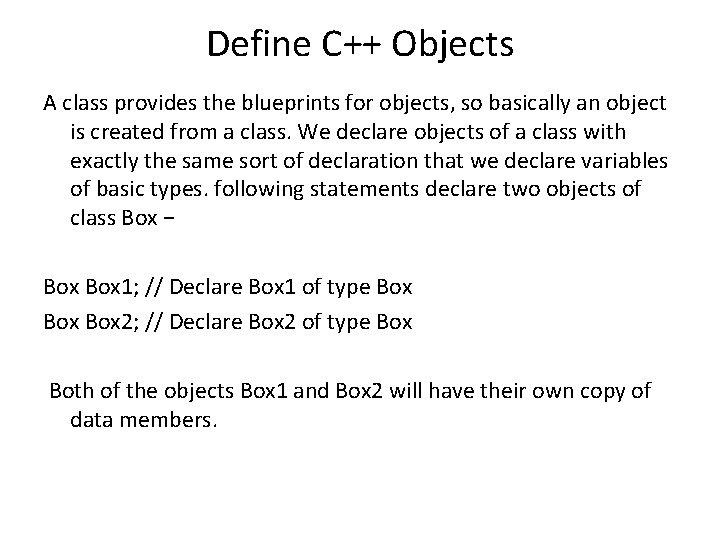Define C++ Objects A class provides the blueprints for objects, so basically an object is created from a class. We declare objects of a class with exactly the same sort of declaration that we declare variables of basic types. following statements declare two objects of class Box − Box 1; // Declare Box 1 of type Box Box 2; // Declare Box 2 of type Box Both of the objects Box 1 and Box 2 will have their own copy of data members.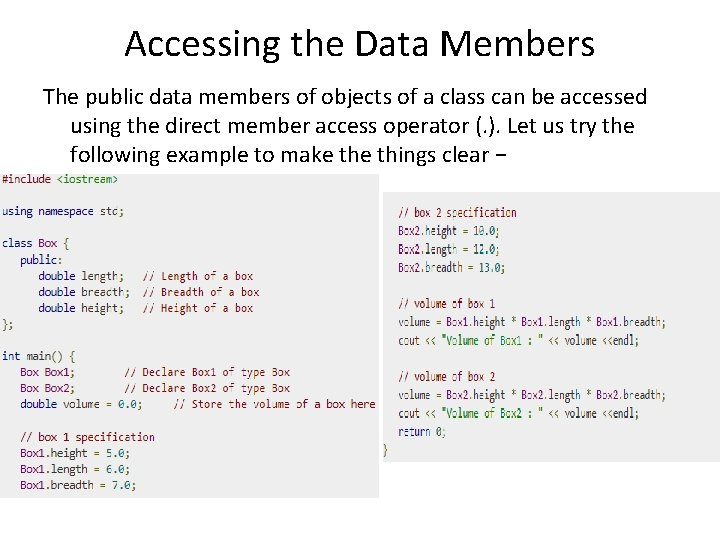Accessing the Data Members The public data members of objects of a class can be accessed using the direct member access operator (. ). Let us try the following example to make things clear −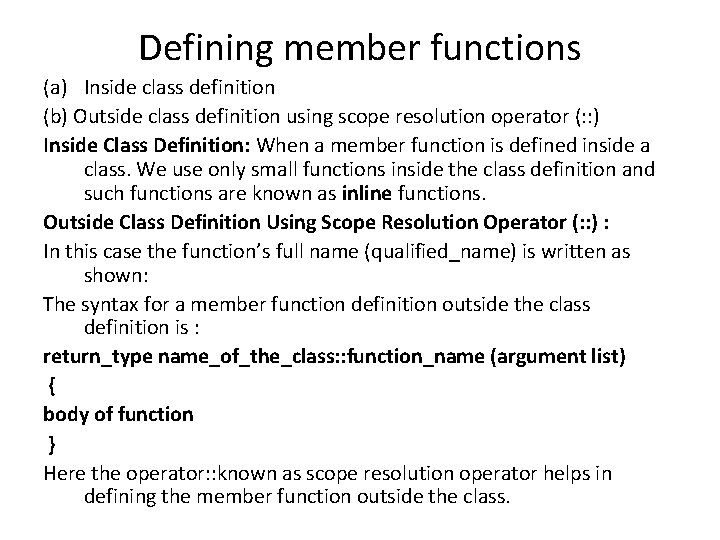Defining member functions (a) Inside class definition (b) Outside class definition using scope resolution operator (: : ) Inside Class Definition: When a member function is defined inside a class. We use only small functions inside the class definition and such functions are known as inline functions. Outside Class Definition Using Scope Resolution Operator (: : ) : In this case the function’s full name (qualified_name) is written as shown: The syntax for a member function definition outside the class definition is : return_type name_of_the_class: : function_name (argument list) { body of function } Here the operator: : known as scope resolution operator helps in defining the member function outside the class.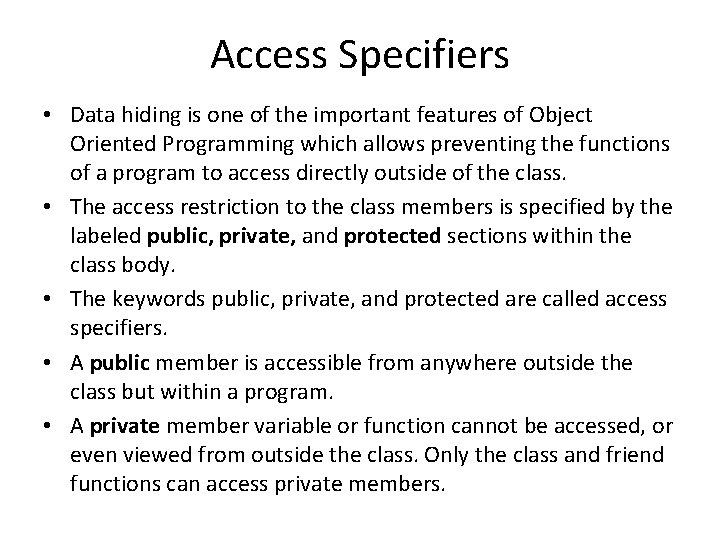Access Specifiers • Data hiding is one of the important features of Object Oriented Programming which allows preventing the functions of a program to access directly outside of the class. • The access restriction to the class members is specified by the labeled public, private, and protected sections within the class body. • The keywords public, private, and protected are called access specifiers. • A public member is accessible from anywhere outside the class but within a program. • A private member variable or function cannot be accessed, or even viewed from outside the class. Only the class and friend functions can access private members.• A protected member variable or function is very similar to a private member but it provided one additional benefit that they can be accessed in child classes which are called derived classes.Static Data Members • A data member of a class can be defined as static. Properties of static member variable are similar to C static variable • It is generally used to store value common to the whole class. • The static data member differs from an ordinary data member in the following ways : (i) Only a single copy of the static data member is used by all the objects. (ii) It can be used within the class but its lifetime is the whole program. (iii) It is initialized to zero when the first object of its class is created. No other initialization is permitted.Class student { static int count; //declaration within class }; • The static data member is defined outside the class as : int student : : count; //definition outside class • The definition outside the class is a must. We can also initialize the static data member at the time of its definition as: int student : : count = 0; If we define three objects as : sudent obj 1, obj 2, obj 3;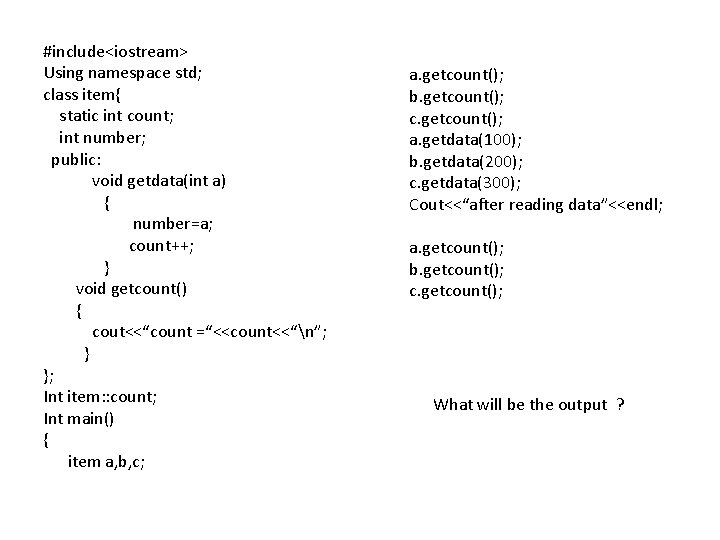#include<iostream> Using namespace std; class item{ static int count; int number; public: void getdata(int a) { number=a; count++; } void getcount() { cout<<“count =“<<count<<“n”; } }; Int item: : count; Int main() { item a, b, c; a. getcount(); b. getcount(); c. getcount(); a. getdata(100); b. getdata(200); c. getdata(300); Cout<<“after reading data”<<endl; a. getcount(); b. getcount(); c. getcount(); What will be the output ?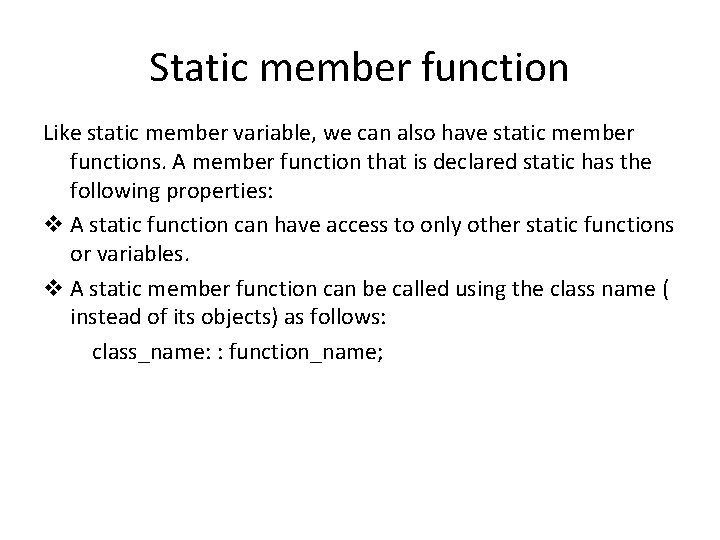Static member function Like static member variable, we can also have static member functions. A member function that is declared static has the following properties: v A static function can have access to only other static functions or variables. v A static member function can be called using the class name ( instead of its objects) as follows: class_name: : function_name;#include<iostream> int item: : count; using namespace std; class Item{ int main() static int count; { int number; item a, b; public: void setnumber() a. setnumber(); { b. setnumber(); number = ++count; Item: : showcount(); } Item c; void shownumber() c. setnumber(); { Item: : showcount(); cout<<“number =“<<number<<“n”; } a. shownumber(); static void showcount() b. shownumber(); { c. shownumber(); cout<<“count: “<<count<<endl; What will be the output } }; cout<<number; error number is not static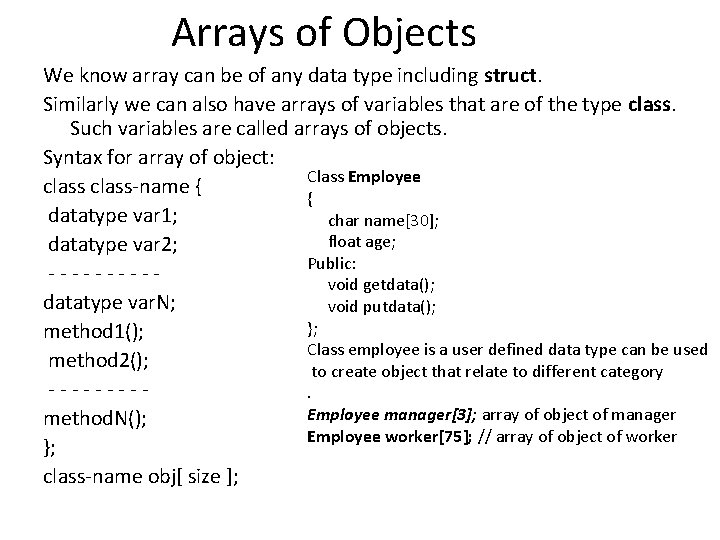Arrays of Objects We know array can be of any data type including struct. Similarly we can also have arrays of variables that are of the type class. Such variables are called arrays of objects. Syntax for array of object: Class Employee class-name { { datatype var 1; char name; float age; datatype var 2; Public: - - - - - void getdata(); datatype var. N; void putdata(); }; method 1(); Class employee is a user defined data type can be used method 2(); to create object that relate to different category - - - - - . Employee manager; array of object of manager method. N(); Employee worker; // array of object of worker }; class-name obj[ size ];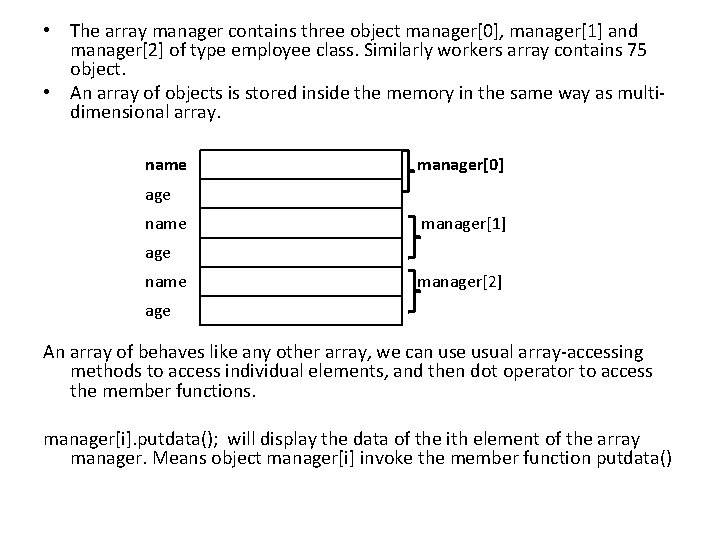• The array manager contains three object manager, manager and manager of type employee class. Similarly workers array contains 75 object. • An array of objects is stored inside the memory in the same way as multidimensional array. name manager age name manager age name manager age An array of behaves like any other array, we can use usual array-accessing methods to access individual elements, and then dot operator to access the member functions. manager[i]. putdata(); will display the data of the ith element of the array manager. Means object manager[i] invoke the member function putdata()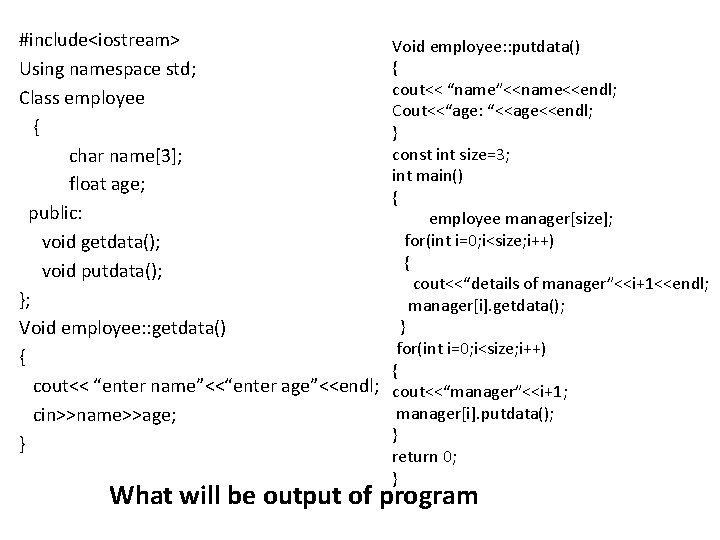#include<iostream> Using namespace std; Class employee { char name; float age; public: void getdata(); void putdata(); }; Void employee: : getdata() { cout<< “enter name”<<“enter age”<<endl; cin>>name>>age; } Void employee: : putdata() { cout<< “name”<<name<<endl; Cout<<“age: “<<age<<endl; } const int size=3; int main() { employee manager[size]; for(int i=0; i<size; i++) { cout<<“details of manager”<<i+1<<endl; manager[i]. getdata(); } for(int i=0; i<size; i++) { cout<<“manager”<<i+1; manager[i]. putdata(); } return 0; } What will be output of programConstructors • A class constructor is a special member function of a class that is executed whenever we create new objects of that class. • A constructor will have exact same name as the class and it does not have any return type at all, not even void. • Constructors can be very useful for setting initial values for certain member variables. • It is called constructor because it constructs the value of data members of the class.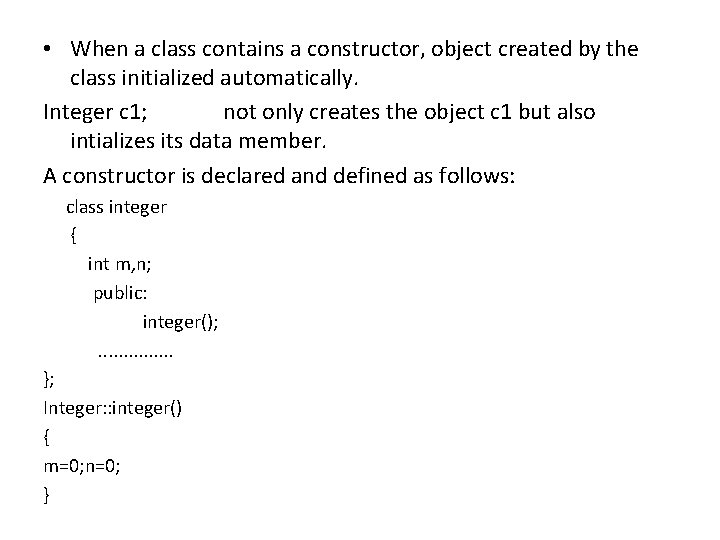• When a class contains a constructor, object created by the class initialized automatically. Integer c 1; not only creates the object c 1 but also intializes its data member. A constructor is declared and defined as follows: class integer { int m, n; public: integer(); . . . . }; Integer: : integer() { m=0; n=0; }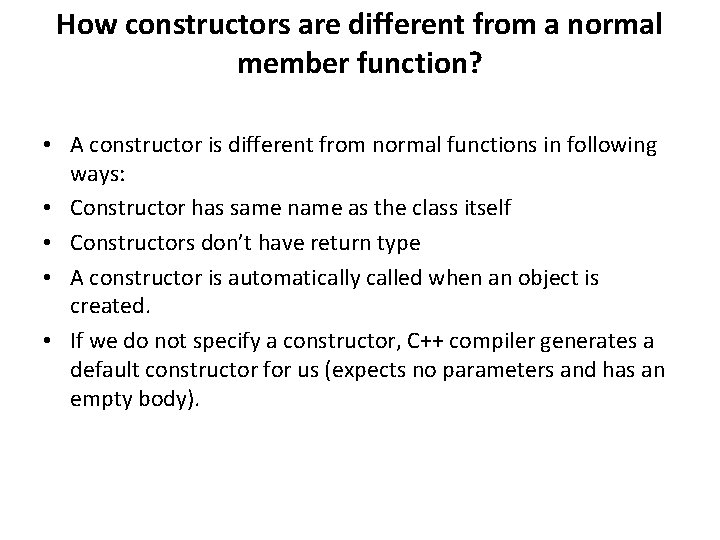How constructors are different from a normal member function? • A constructor is different from normal functions in following ways: • Constructor has same name as the class itself • Constructors don’t have return type • A constructor is automatically called when an object is created. • If we do not specify a constructor, C++ compiler generates a default constructor for us (expects no parameters and has an empty body).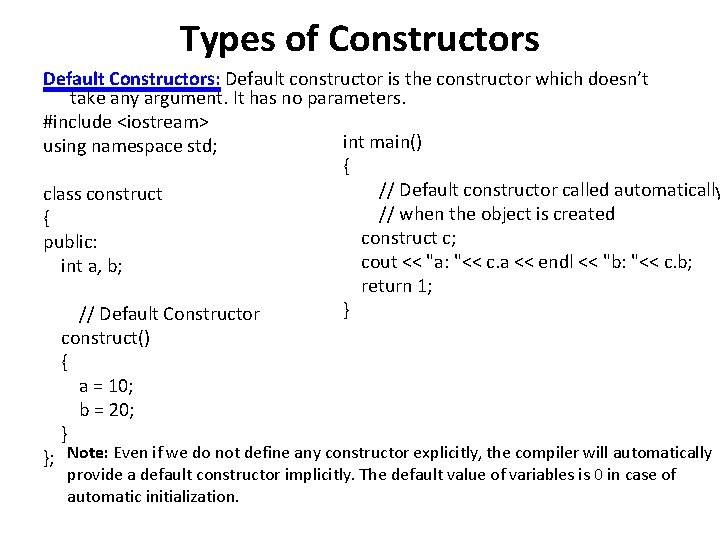Types of Constructors Default Constructors: Default constructor is the constructor which doesn’t take any argument. It has no parameters. #include <iostream> int main() using namespace std; { // Default constructor called automatically class construct // when the object is created { construct c; public: cout << "a: "<< c. a << endl << "b: "<< c. b; int a, b; return 1; } // Default Constructor construct() { a = 10; b = 20; } }; Note: Even if we do not define any constructor explicitly, the compiler will automatically provide a default constructor implicitly. The default value of variables is 0 in case of automatic initialization.#include <iostream> using namespace std; class Test { static int x; public: Test() { x++; } static int get. X() {return x; } }; int Test: : x = 0; int main() { cout << Test: : get. X() << " "; Test t; cout << Test: : get. X(); } What will be the output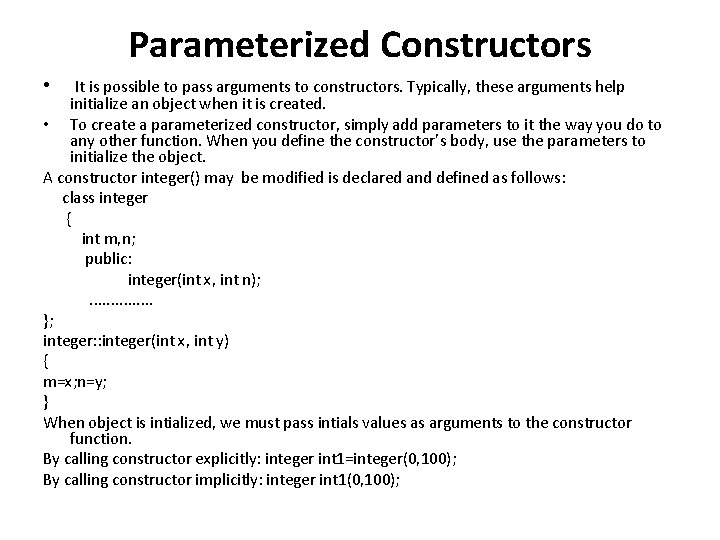Parameterized Constructors • It is possible to pass arguments to constructors. Typically, these arguments help initialize an object when it is created. • To create a parameterized constructor, simply add parameters to it the way you do to any other function. When you define the constructor’s body, use the parameters to initialize the object. A constructor integer() may be modified is declared and defined as follows: class integer { int m, n; public: integer(int x, int n); . . . . }; integer: : integer(int x, int y) { m=x; n=y; } When object is intialized, we must pass intials values as arguments to the constructor function. By calling constructor explicitly: integer int 1=integer(0, 100); By calling constructor implicitly: integer int 1(0, 100);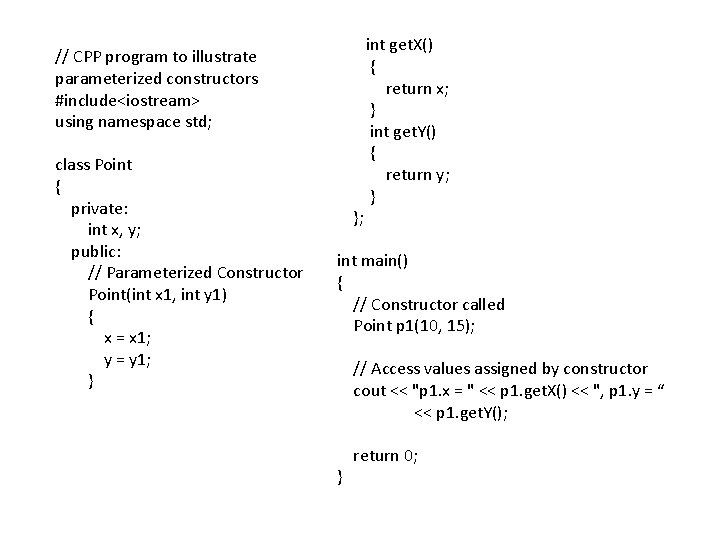// CPP program to illustrate parameterized constructors #include<iostream> using namespace std; class Point { private: int x, y; public: // Parameterized Constructor Point(int x 1, int y 1) { x = x 1; y = y 1; } int get. X() { return x; } int get. Y() { return y; } }; int main() { // Constructor called Point p 1(10, 15); // Access values assigned by constructor cout << "p 1. x = " << p 1. get. X() << ", p 1. y = “ << p 1. get. Y(); return 0; }Copy Constructor • A copy constructor is a member function which initializes an object using another object of the same class. • A copy constructor has the following general function prototype: Class. Name (const Class. Name &old_obj); Following is a simple example of copy constructor. int main() #include<iostream> using namespace std; { Point p 1(10, 15); class Point p 2 = p 1; { private: // Let us access values assigned by constructors int x, y; cout << "p 1. x = " << p 1. get. X() << ", p 1. y = “ public: << p 1. get. Y(); Point(int x 1, int y 1) { x = x 1; y = y 1; } cout << "np 2. x = " << p 2. get. X() << ", p 2. y = “ << p 2. get. Y(); // Copy constructor Point(const Point &p 2) {x = p 2. x; y = p 2. y; } return 0; } int get. X() { return x; } int get. Y() { return y; } };When is copy constructor called? In C++, a Copy Constructor may be called in following cases: 1. When an object of the class is returned by value. 2. When an object of the class is passed (to a function) by value as an argument. 3. When an object is constructed based on another object of the same class. 4. When compiler generates a temporary object.Destructors in C++ • What is destructor? Destructor is a member function which destructs or deletes an object. • When is destructor called? A destructor function is called automatically when the object goes out of scope: (1) the function ends (2) the program ends (3) a block containing local variables ends (4) a delete operator is called • How destructors are different from a normal member function? Destructors have same name as the class preceded by a tilde (~) Destructors don’t take any argument and don’t return anythingclass String { Can there be more than one destructor in a class? private: No, there can only one destructor in a class with classname char *s; preceded by ~, no parameters and no return type. int size; public: String(char *); // constructor ~String(); // destructor }; String: : String(char *c) { size = strlen(c); s = new char[size+1]; strcpy(s, c); } String: : ~String() { delete []s; }